International
Tables for
Crystallography
Volume A
Space-group symmetry
Edited by Th. Hahn

International Tables for Crystallography (2006). Vol. A, ch. 8.1, p. 722

Section 8.1.3. Symmetry operations and symmetry groups

H. Wondratscheka*

aInstitut für Kristallographie, Universität, D-76128 Karlsruhe, Germany
Correspondence e-mail: hans.wondratschek@physik.uni-karlsruhe.de

8.1.3. Symmetry operations and symmetry groups

| top | pdf |

Definition: A symmetry operation of a given object in point spaceis a motion ofwhich maps this object (point, set of points, crystal pattern etc.) onto itself.

Remark: Any motion may be a symmetry operation, because for any motion one can construct an object which is mapped onto itself by this motion.

For the set of all symmetry operations of a given object, the following relations hold:

 (a) successive application of two symmetry operations of an object results in a third symmetry operation of that object; (b) the inverse of a symmetry operation is also a symmetry operation; (c) there exists an identity operation'which leaves each point of the space fixed: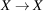. This operationis described (in any coordinate system) by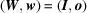or by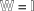and it is a symmetry operation of any object. (d) The associative law'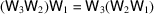is valid.

One can show, however, that in general the `commutative law'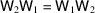is not obeyed for symmetry operations.

The properties (a) to (d) are the group axioms. Thus, the set of all symmetry operations of an object forms a group, the symmetry group of the object or its symmetry. The mathematical theorems of group theory, therefore, may be applied to the symmetries of objects.

So far, only rather general objects have been considered. Crystallographers, however, are particularly interested in the symmetries of crystals. In order to introduce the concept of crystallographic symmetry operations, crystal structures, crystal patterns and lattices have to be taken into consideration. This will be done in the following section.